# Test Prep Plan - Take a practice test

Take this practice test to check your existing knowledge of the course material. We'll review your answers and create a Test Prep Plan for you based on your results.
How Test Prep Plans work
1
2Based on your results, we'll create a customized Test Prep Plan just for you!
3Study smarter
Study more effectively: skip concepts you already know and focus on what you still need to learn.

# NES Math: Graphing Derivatives Chapter Exam

Exam Instructions:

Choose your answers to the questions and click 'Next' to see the next set of questions. You can skip questions if you would like and come back to them later with the yellow "Go To First Skipped Question" button. When you have completed the practice exam, a green submit button will appear. Click it to see your results. Good luck!

### Page 1

#### Question 1 1. What is the equation of the line normal to f(x) at the point x=1?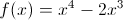#### Question 2 2. Which could be the graph of the derivative of the function below?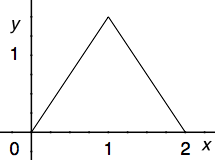#### Question 3 3. What is the equation of the line tangent to f(x) at the point x=0?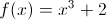#### Question 4 4. The graph shown is an example of a function that: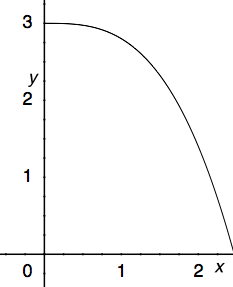### Page 2

#### Question 6 6. The graph shown is an example of a function that: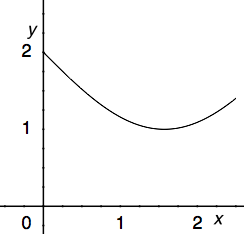#### Question 7 7. What is the slope of the tangent to r(s) at s = 0?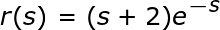#### Question 8 8. What is the slope of the line normal to f(x) at x=1?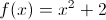#### Question 9 9. What is the global minimum for the function y=f(x) below between x=0 and x=2?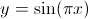#### Question 10 10. What is the slope of a line normal to f(x)?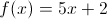### Page 3

#### Question 11 11. Consider the function g(x) below. At x = 0 is this function concave up, concave down, or an inflection point?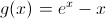#### Question 12 12. Which of the following BEST describes point B?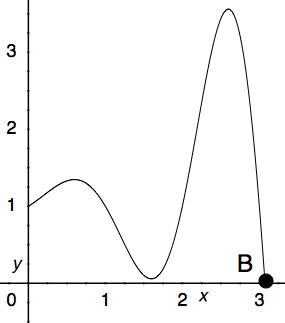#### Question 13 13. What is value of y at the finite local maximum for the function y=f(x) below?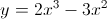#### Question 14 14. The derivative of the following graph is _____ at point A.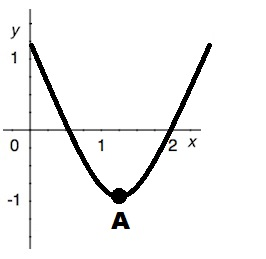#### Question 15 15. The derivative of the following graph is _____ at point D.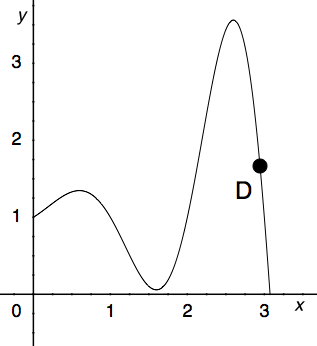### Page 4

#### Question 16 16. Over the region shown, the global minimum is at the point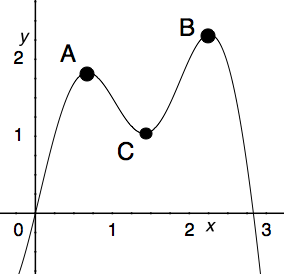#### Question 18 18. Which of the following BEST describes point A?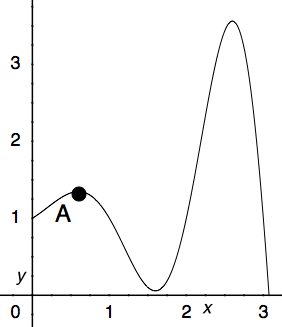#### Question 20 20. Where is the global maximum for the function y=f(x) below between x=0 and x=2?### Page 5

#### Question 21 21. Which of the following is a local minimum?#### Question 22 22. The graph shown is an example of a function that: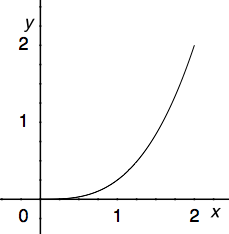#### Question 24 24. The derivative of the following graph is _____ at point C.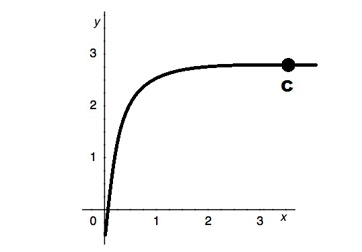#### Question 25 25. Between x=1 and x=3, the following function _____.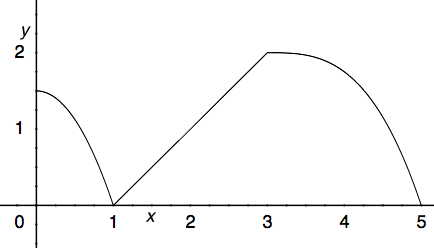### Page 6

#### Question 26 26. Which of the following BEST describes point C?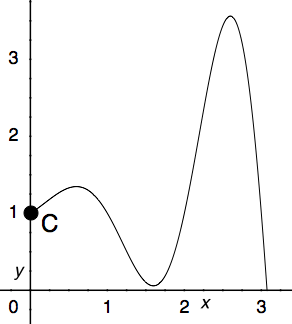#### Question 27 27. Over the region shown, point A is known as _____.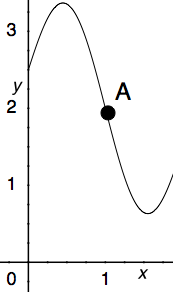#### Question 28 28. What is the global minimum for the function y=f(x) below between x=0 and x=2?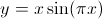#### Question 29 29. Consider the function f(x) below. At x = 1 is this function concave up, concave down, or an inflection point?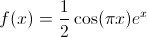#### NES Math: Graphing Derivatives Chapter Exam Instructions

Choose your answers to the questions and click 'Next' to see the next set of questions. You can skip questions if you would like and come back to them later with the yellow "Go To First Skipped Question" button. When you have completed the practice exam, a green submit button will appear. Click it to see your results. Good luck!

Support